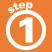Home    |    Teacher    |    Parents    |    Glossary    |    About UsLike fractions are fractions with the same denominator. You can add and subtract like fractions easily - simply add or subtract the numerators and write the sum over the common denominator.

Before you can add or subtract fractions with different denominators, you must first find equivalent fractions with the same denominator, like this:

1. Find the smallest multiple (LCM) of both numbers.
2. Rewrite the fractions as equivalent fractions with the LCM as the denominator.

When working with fractions, the LCM is called the least common denominator (LCD). Click on the examples below.

 Homework Help | Pre-Algebra | FractionsEmail this page to a friendSearch·  Definitions·  Reducing fractions·  Adding and subtract-     ing fractions·  Multiplying fractions·  Dividing fractions·  Adding and subtract-     ing mixed numbers·  Multiplying     mixed numbers·  Dividing     mixed numbersFirst Glance In Depth Examples WorkoutAdding and subtracting fractions